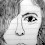Latest Articles

# Finding Squares of Numbers by Vedic Maths Method

Info Post

Definition : Whatever the deficiency subtract that deficit from the number and write along side the square of that deficit

We have placed '0' before 36 as the final output should be a six-digit number . The above method is similar for numbers like 96 2 , 9988 2 , etc...

In case of Surplus , the above sutra sounds true for any numbers close to the powers of 10

Carrying over is done for each value of hundredth place value except for '0'. The above method is similar for numbers like 106 2 , 908 , etc...

Algebric Proof :

Let 'x' be the base  and 'y' be the deficit .The numbers near or less than power of "10" can be treated as ( x - y ).

That is , 9  = ( 10 - 1)
994 = ( 1000 - 6 )
96 = ( 100 - 4 ) , etc...

( x - y ) 2 =  x 2 - 2 x y + y 2
= x ( x - 2y) + y 2
= x [ ( x - y ) - y ] + y 2
= base [ (number) - deficiency ] + deficit2
Thus ,
985 2
which can be written as  ( 1000 - 15 )
(or) = 1000 [ ( 985 - 15 )] + 15 2
= 970000 + 225
= 970225
Therefore , 985 = 970225

Simple and Effective Trick for BASE 50

Share:

1.